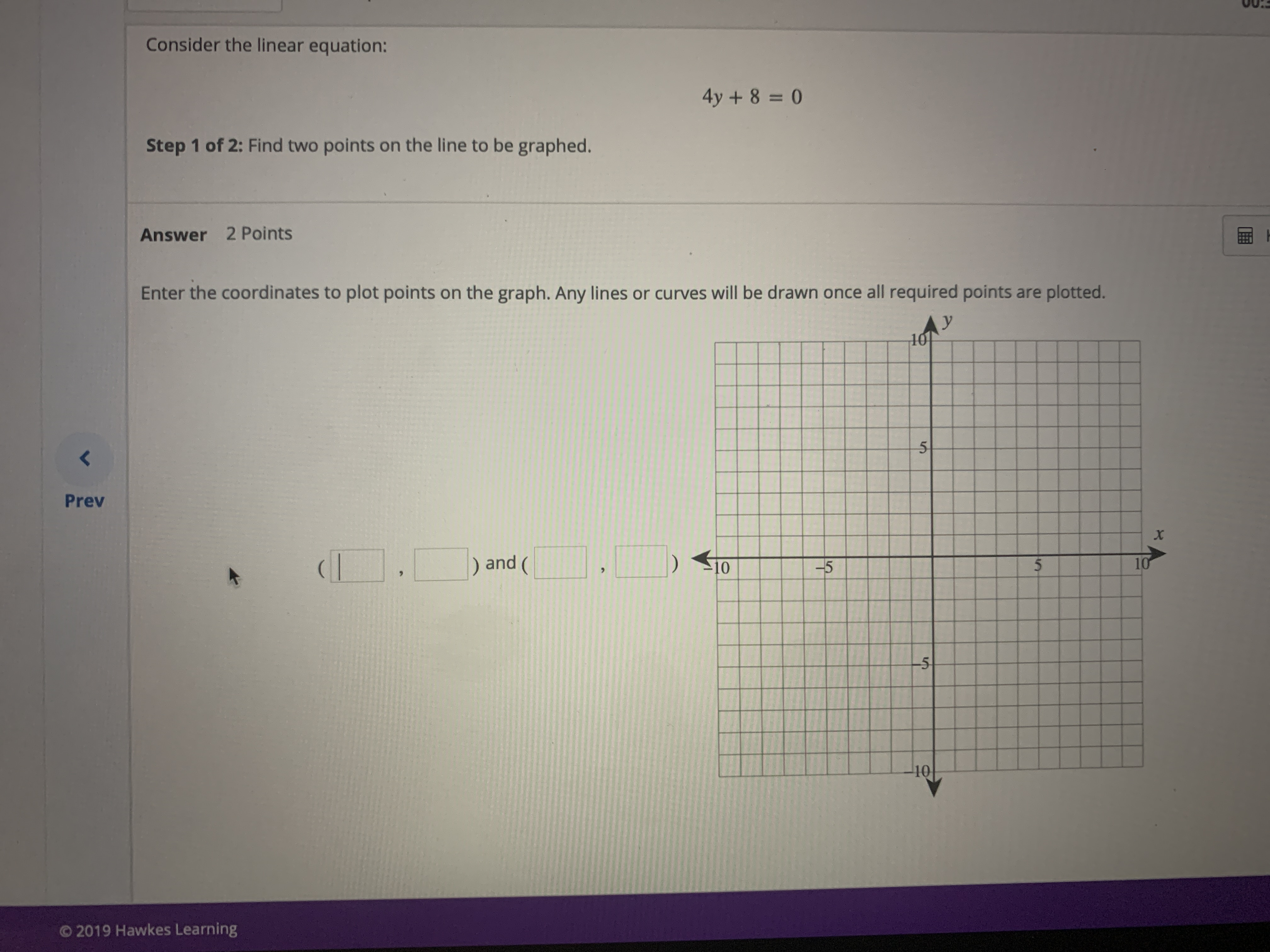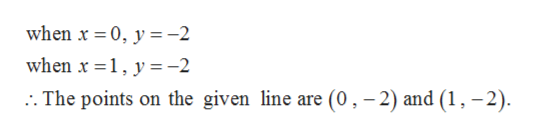Consider the linear equation:4y+8=0Step 1 of 2: Find two points on the line to be graphed.Answer 2 PointsEnter the coordinates to plot points on the graph. Any lines or curves will be drawn once all required pointsare plotted.y105PrevXand (105-5(10510O2019 Hawkes Learning

Questionhelp_outlineImage TranscriptioncloseConsider the linear equation: 4y+8=0 Step 1 of 2: Find two points on the line to be graphed. Answer 2 Points Enter the coordinates to plot points on the graph. Any lines or curves will be drawn once all required points are plotted. y 10 5 Prev X and ( 10 5 -5 ( 10 5 10 O2019 Hawkes Learning fullscreen
Step 1

Given,

Step 2

On simplification, we get

Step 3

Therefore, for any value of x, the value ...help_outlineImage Transcriptionclosewhen x 0, y-2 when x 1, y-2 The points on the given line are (0, -2) and (1,-2) fullscreen

Want to see the full answer?

See Solution

Want to see this answer and more?

Our solutions are written by experts, many with advanced degrees, and available 24/7

See Solution
Tagged in

Other